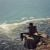# Ross Honsberger

Updated on
Covid-19
 Name  Ross HonsbergerRole  Author Education  University of Toronto Books  More Mathematical Morsels, Ingenuity in mathematics, Episodes in Nineteent, From Erdos to Kiev, Mathematical gems

## Susan kayne with ross honsberger at the big e draft horse show

Ross Honsberger (1929–2016) was a Canadian mathematician and author on recreational mathematics.

## Life

Honsberger studied mathematics at the University of Toronto, with a bachelor's degree, and then worked for ten years as a teacher in Toronto, before continuing his studies at the University of Waterloo (master's degree). Since 1964 he had been on the faculty of mathematics, where he later became a professor emeritus. He dealt with combinatorics and optimization, especially with mathematics education. He developed education courses, for example, on combinatorial geometry, held frequently lectures for students and math teachers, and was editor of the Ontario Secondary School Mathematics Bulletin. He wrote numerous books on elementary mathematics (geometry, number theory, combinatorics, probability theory), and recreational mathematics (often at the Mathematical Association of America, MAA), with him in his own words using the book by Hans Rademacher and Otto Toeplitz of numbers and figures as a model. Frequent were his expositions of problems at the International Mathematical Olympiads and other competitions.

Edsger W. Dijkstra called his Mathematical Gems "delightful".

## Books

• Ingenuity in Mathematics, New Mathematical Library, Random House / Singer 1970
• Mathematical Gems, MAA 1973, 2003 (Mathematical Expositions Dolciani Vol.1), German Mathematical gems of elementary combinatorics, number theory and geometry, Wiley, 1990, ISBN 3-528-08474-X, Chapter "The Story of Louis Posa".
• Mathematical Gems 2, MAA 1975 (Vol.2 Dolciani Mathematical Expositions)
• Mathematical Gems 3, MAA 1985, 1991 (vol.9 Dolciani Mathematical Expositions)
• Mathematical Morsels, MAA 1978 (Vol.3 Dolciani Mathematical Expositions)
• More Mathematical Morsels, MAA 1991 (Dolciani Bd.10 Mathematical Expositions)
• Mathematical Plums, MAA 1979 (vol.4 Dolciani Mathematical Expositions)
• Mathematical Chestnuts from around the world, MAA 2001 (Dolciani Bd.24 Mathematical Expositions)
• Mathematical Diamonds, MAA 2003
• In Polya's Footsteps, MAA 1997 (Dolciani Bd.19 Mathematical Expositions)
• Episodes in nineteenth and twentieth century euclidean geometry, MAA 1995
• From Erdos to Kiev - Problems of Olympiad Caliber, MAA 1997
• Mathematical Delights, MAA 2004 (Dolciani Mathematics Expositions Bd.28)
• ## References

Similar Topics
The Clown (1916 film)
Odile Ahouanwanou
Daniel Caspary
Topics

 B i Link H2
 L